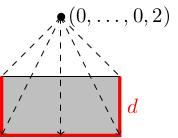# 4.03 CW complexes and the HEP

Below the video you will find accompanying notes and some pre-class questions.

# Notes

## CW pairs have the homotopy extension property

(0.00) If $$X$$ is a CW complex and $$A$$ is a closed subcomplex then the pair $$(X,A)$$ has the HEP.

(0.30) A closed subcomplex is a union of closed cells of $$X$$ such that $$X$$ is obtained by adding cells to $$A$$. The pair $$(X,A)$$ (where $$X$$ is a CW complex and $$A$$ is a closed subcomplex) is sometimes called a CW pair.

(1.18) If $$e$$ is a $$k$$-dimensional disc then there is a continuous map $$e\times[0,1]\to(\partial e\times[0,1])\cup e\times\{0\}$$.
Below is a picture of the map in the case $$k=1$$. If we embed $$e\times[0,1]$$ into $$\mathbf{R}^{k+1}$$ as the subset $\{(x,y)\in\mathbf{R}^k\times\mathbf{R}\ :\ |x|\leq 1,\ y\in[0,1]\}$ then the linear projection from the point $$(0,\ldots,0,2)$$ to the indicated subset ($$d$$, in red) of the boundary $$\partial(e\times[0,1])$$ is the required map.(4.16) If $$X$$ is a space obtained from $$A$$ by attaching a single $$k$$-cell $$e$$ then the pair $$(X,A)$$ has the HEP.
(4.58) Recall that the HEP states that if we are given a function $$F\colon X\to Y$$ and a homotopy $$h_t\colon A\to A$$ of $$h_0=F|_A$$ then there is a homotopy $$H_t\colon X\to X$$ of $$H_0=F$$ such that $$(H_t)|_A=h_t$$. Recall that $$H_t(x)$$ means $$H(x,t)$$ for some continuous map $$H\colon X\times[0,1]\to Y$$.

(6.38) In our case, $$X=A\cup_{\varphi}e$$ for some attaching map $$\varphi\colon \partial e\to A$$; we have $$H$$ already defined on $$A\times[0,1]$$ (where it is equal to $$h$$) and on $$e\times\{0\}$$ (where it is equal to $$F$$) and we want to define the homotopy $$H$$ on $$X\times[0,1]$$.

(7.50) The only points where we do not already know $$H$$ are the points of $$e\times[0,1]\subset X\times[0,1]$$. If we let $$G\colon e\times[0,1]\to(\partial e\times[0,1])\cup e\times\{0\}$$ be the map from the previous lemma then we can define $$H|_{e\times[0,1]}$$ to be the composition $$H\circ G$$: $$G$$ takes points of $$e\times[0,1]$$ into the region $$(\partial e\times[0,1])\cup e\times\{0\}$$, which is attached to $$(A\times[0,1])\cup e\times\{0\}$$, where we already know how to define $$H$$, so this circular-looking definition actually makes sense.

(10.30) The theorem now follows by induction: $$X$$ is obtained from $$A$$ by attaching cells, and each time you attach a cell you can extend a homotopy from $$A$$ over the new cell.

## 1-dimensional CW complexes

(11.03) Any connected 1-dimensional CW complex $$X$$ is homotopy equivalent to a wedge of (possibly infinitely many) circles (in other words, a single 0-cell with a bunch of 1-cells attached).
(12.01) We need to find a contractible subcomplex $$A$$ containing all the 0-cells: by the previous theorem, $$(X,A)$$ has the HEP. By the theorem we proved last time, this means that $$X\simeq X/A$$, and $$X/A$$ is a CW complex with a single 0-cell (the image of $$A$$ under the quotient map) and a bunch of 1-cells (the 1-cells not contained in $$A$$).

(13.17) To find'' a contractible subcomplex, we partially order the set of contractible subcomplexes by inclusion. By Zorn's lemma, there is a contractible subcomplex which is maximal with respect to this partial order (i.e. one which is not contained in any bigger contractible subcomplex). There may be many choices of maximal contractible subcomplex.

(14.56) A maximal contractible subcomplex $$A$$ necessarily passes through all the 0-cells: otherwise we could add an edge connecting $$A$$ to a 0-cell it does not contain to obtain a bigger contractible subcomplex.

(16.26) I'm taking Zorn's lemma as an axiom here: it's equivalent to another axiom of set theory known as the Axiom of Choice. This is the assumption that you can make infinitely many choices simultaneously (more precisely, that an infinite product of nonempty sets is nonempty). You could think of our proof as saying: start with a single edge and start adding more edges to get a maximal contractible subcomplex; you have to make infinitely many choices along the way, and there's no algorithm for doing this if you don't know anything more about your CW complex. Therefore we just assume we can make these choices. This is a non-constructive proof (we don't give an algorithm for making the choices) and some mathematicians, logicians and philosophers find this kind of argument troubling.

# Pre-class questions

1. Look back at the argument that any 1-dimensional connected CW complex is homotopy equivalent to a wedge of circles. Where did we use the fact that it is connected?